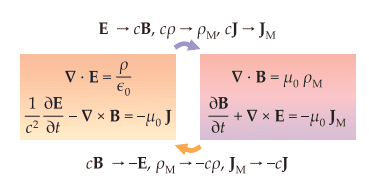# About Maxwell's equations and the attempt to insert a magnetic monopole

LCSphysicist
I was reading somethings about magnetic monopoles, and how, if it were discovered its existence, it would changes the Maxwell equations, in summarizing, is not the first time i see this:It is the consequences of the existence of a magnetic monopole? That is, IF it exist, SO the imagem is true. Or is just a possible, and not exactly truth, way to imagine how the Maxwell Equations would suffer under such changes?

•